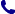• Kontakt
• Newsletter Zapisz się po informacje o nowościach i specjalnych ofertach. Administrator danych: ABE-IPS sp. z o.o.
Wybierz interesujące Cię kategorie
Wyrażam zgodę na przetwarzanie moich danych osobowych w celach marketingowych, tym samym akceptuję Regulamin Newslettera i Politykę prywatności.
Wyrażam zgodę na przesyłanie informacji handlowych drogą elektroniczną.
Wypisz się
• Klienci instytucjonalni
• Dostawa
• O nas
Dostęp on-line

# Książki

0.00 PLN
Schowek (0)
 Schowek jest pusty

## Introduction to Partial Differential Equations

 Autorzy Wydawnictwo Springer, Berlin Data wydania Liczba stron 283 Forma publikacji książka w twardej oprawie Język angielski ISBN 9783319489346 Kategorie Rachunek różniczkowy i formuły
369.60 PLN (z VAT)
\$83.14 / €79.24 / £68.79 /
Produkt na zamówienie
Dostawa 5-6 tygodni
Ilość
Do schowka

## Opis książki

This modern take on partial differential equations does not require knowledge beyond vector calculus and linear algebra. The author focuses on the most important classical partial differential equations, including conservation equations and their characteristics, the wave equation, the heat equation, function spaces, and Fourier series, drawing on tools from analysis only as they arise.
Within each section the author creates a narrative that answers the five questions:
1. What is the scientific problem we are trying to understand?
2. How do we model that with PDE?
3. What techniques can we use to analyze the PDE?
4. How do those techniques apply to this equation?
5. What information or insight did we obtain by developing and analyzing the PDE?
The text stresses the interplay between modeling and mathematical analysis, providing a thorough source of problems and an inspiration for the development of methods.

Introduction to Partial Differential Equations

## Spis treści

1. Introduction.- 2. Preliminaries.- 3. Conservation Equations and Characteristics.- 4. The Wave Equation.- 5. Separation of Variables.- 6. The Heat Equation.- 7. Function Spaces.- 8. Fourier Series.- 9. Maximum Principles.- 10. Weak Solutions.- 11. Variational Methods.- 12. Distributions.- 13. The Fourier Transform.- A. Appendix: Analysis Foundations.- References.- Notation Guide.- Index.

## Polecamy również książki801 777 223